### Showing videos fromEddie Woo with a total of 4,235 videos02:11
Nominate someone for the 2021 Australian of the Year Awards!
1D11h ago09:05
Two-Way Tables (2 of 2: Michael Jordan vs. Reggie Miller)
2D17h ago09:14
Cumulative Frequency (1 of 2: Basic calculations)
6D17h ago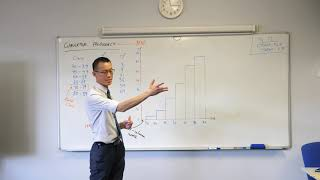11:23
Cumulative Frequency (2 of 2: Constructing & using an ogive)
8D17h ago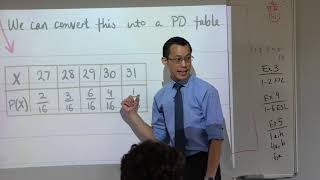03:29
What's the connection between Arithmetic Mean & Expected Value?
10D17h ago09:55
Comparing Measures of Central Tendency (2 of 2: Median)
12D17h ago08:33
Comparing Measures of Central Tendency (1 of 2: Mean & Mode)
14D11h ago11:41
Phenomenom: Eddie Woo and the Fun Guys (who are actually quite serious)
27D17h ago07:44
Differentiating Exponentials with Other Bases (3 of 3: Establishing the formula)
1m2D ago09:57
Differentiating Exponentials with Other Bases (2 of 3: Deriving a key result)
1m4D ago10:05
Differentiating Exponentials with Other Bases (1 of 3: Review questions)
1m6D ago24:54
Q&A at Sydney University | Arts & Social Sciences Livestream
1m8D ago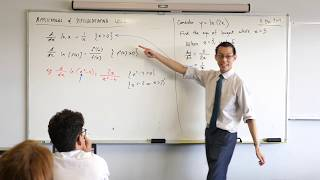11:46
Differentiating the Logarithmic Function (5 of 5: Equation of a tangent)
1m10D ago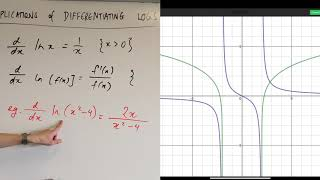07:54
Differentiating the Logarithmic Function (4 of 5: Restricting domain)
1m12D ago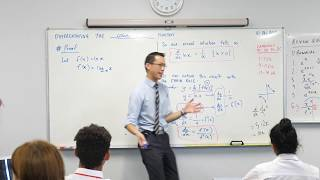05:41
Differentiating the Logarithmic Function (3 of 5: Proof by implicit differentiation)
1m14D ago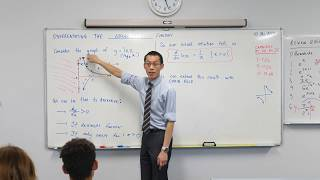08:36
Differentiating the Logarithmic Function (2 of 5: Incorporating chain rule)
1m16D ago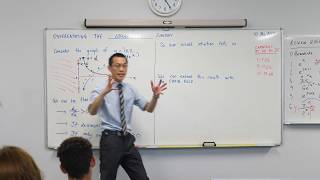11:38
Differentiating the Logarithmic Function (1 of 5: Using visual intuition)
1m18D ago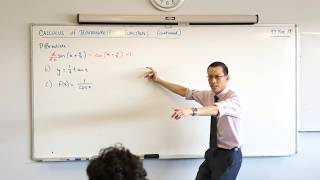09:43
Differentiating Simple Trigonometric Functions (examples)
1m28D ago00:24
Eddie Woo's Magical Maths - available through Booktopia!
2m1D ago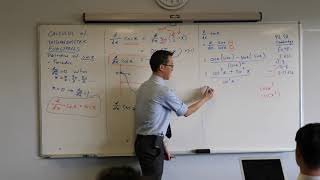04:15
Intro to Trigonometric Calculus (5 of 5: Derivative of tan x)
2m3D ago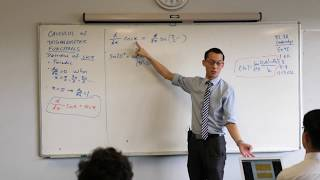07:45
Intro to Trigonometric Calculus (4 of 5: Derivative of cos x)
2m5D ago08:20
Intro to Trigonometric Calculus (3 of 5: Investigating gradient magnitude)
2m7D ago08:21
Intro to Trigonometric Calculus (2 of 5: Investigating the gradient of sin x)
2m9D ago04:52
Intro to Trigonometric Calculus (1 of 5: Review questions)
2m10D ago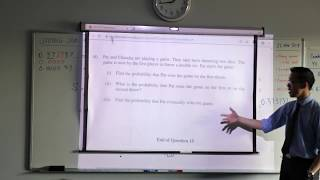10:18
Limiting Sum Applications (3 of 3: Probability problem)
2m13D ago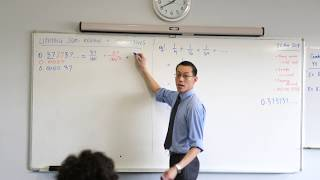08:40
Limiting Sum Applications (2 of 3: Visual interpretation & recurring decimals)
2m14D ago08:52
Limiting Sum Applications (1 of 3: Derivation of formula)
2m15D ago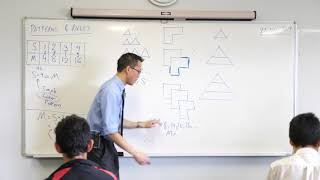03:41
Patterns & Rules (2 of 2: Unpacking the algebraic expressions)
2m16D ago12:55
Patterns & Rules (1 of 2: Building geometric shapes)
2m17D ago07:35
International Time Zones (2 of 2: How do we use them?)
2m18D ago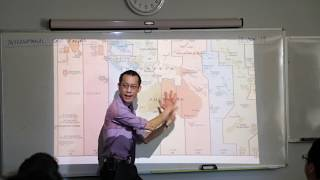08:14
International Time Zones (1 of 2: Why do we need them?)
2m19D ago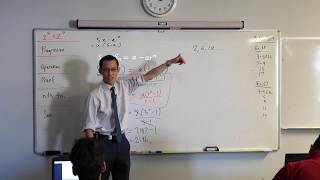07:12
Intro to Geometric Progressions (3 of 3: Different versions of sum formula)
2m20D ago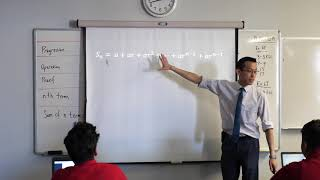07:45
Intro to Geometric Progressions (2 of 3: Algebraic derivation of sum formula)
2m22D ago08:03
Intro to Geometric Progressions (1 of 3: Definitions)
2m23D ago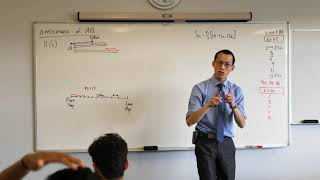07:09
Sum of an Arithmetic Progression (5 of 5: How far between the truck stops?)
2m25D ago06:46
Sum of an Arithmetic Progression (4 of 5: Application to truck problem)
2m26D ago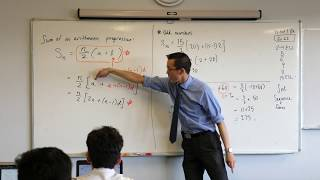04:02
Sum of an Arithmetic Progression (3 of 5: Determining series length)
2m27D ago06:55
Sum of an Arithmetic Progression (2 of 5: Developing & using alternate formula)
2m28D ago06:56
Sum of an Arithmetic Progression (1 of 5: Visual derivation of formula)
2m29D ago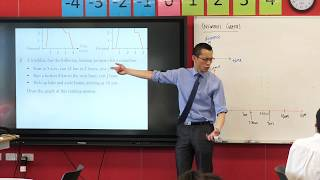07:57
Informal Graphs (2 of 2: Interpretation & construction)
2m30D ago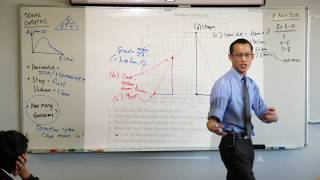03:59
Travel Graphs (2 of 2: Interpreting & making calculations)
3m1D ago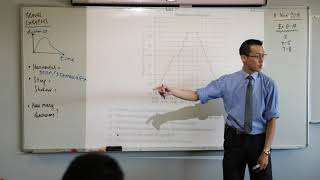09:42
Travel Graphs (1 of 2: Overview & introductory example)
3m2D ago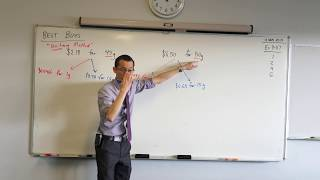05:14
Calculating Best Buys (how to use two comparable methods)
3m3D ago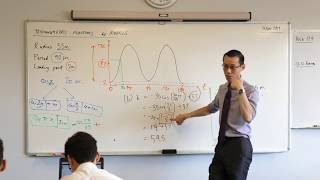08:05
The Ferris Wheel - Trigonometric Function Model (3 of 3: Interpreting results)
3m4D ago09:13
The Ferris Wheel - Trigonometric Function Model (2 of 3: Adjusting the period)
3m5D ago09:59
The Ferris Wheel - Trigonometric Function Model (1 of 3: Setting up the equation)
3m6D ago10:09
3m7D ago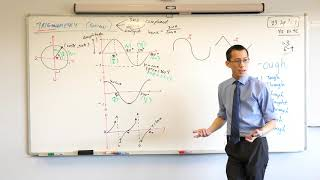08:35
Reviewing Trigonometric Functions (3 of 3: Basic features of the tan graph)
3m9D ago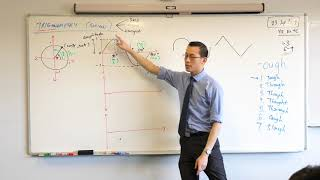08:47
Reviewing Trigonometric Functions (2 of 3: Features of sine and cosine)
3m10D ago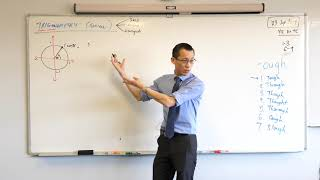12:13
Reviewing Trigonometric Functions (1 of 3: Re-introducing the sine graph)
3m11D ago06:01
Solving Equation Problems (3 of 3: Translating to an equation)
3m13D ago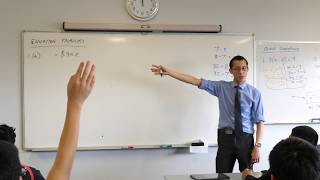06:48
Solving Equation Problems (2 of 3: Using inverses to change the subject)
3m14D ago08:39
Solving Equation Problems (1 of 3: Reviewing abstract equations)
3m15D ago